This book is archived and will be removed July 6, 2022. Please use the updated version.

The Nature of Light

# 7 Huygens’s Principle

### Learning Objectives

By the end of this section, you will be able to:

• Describe Huygens’s principle
• Use Huygens’s principle to explain the law of reflection
• Use Huygens’s principle to explain the law of refraction
• Use Huygens’s principle to explain diffraction

So far in this chapter, we have been discussing optical phenomena using the ray model of light. However, some phenomena require analysis and explanations based on the wave characteristics of light. This is particularly true when the wavelength is not negligible compared to the dimensions of an optical device, such as a slit in the case of diffraction. Huygens’s principle is an indispensable tool for this analysis.

(Figure) shows how a transverse wave looks as viewed from above and from the side. A light wave can be imagined to propagate like this, although we do not actually see it wiggling through space. From above, we view the wave fronts (or wave crests) as if we were looking down on ocean waves. The side view would be a graph of the electric or magnetic field. The view from above is perhaps more useful in developing concepts about wave optics.

A transverse wave, such as an electromagnetic light wave, as viewed from above and from the side. The direction of propagation is perpendicular to the wave fronts (or wave crests) and is represented by a ray.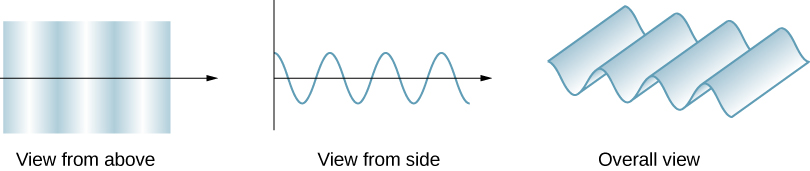The Dutch scientist Christiaan Huygens (1629–1695) developed a useful technique for determining in detail how and where waves propagate. Starting from some known position, Huygens’s principle states that every point on a wave front is a source of wavelets that spread out in the forward direction at the same speed as the wave itself. The new wave front is tangent to all of the wavelets.

(Figure) shows how Huygens’s principle is applied. A wave front is the long edge that moves, for example, with the crest or the trough. Each point on the wave front emits a semicircular wave that moves at the propagation speed v. We can draw these wavelets at a time t later, so that they have moved a distance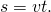The new wave front is a plane tangent to the wavelets and is where we would expect the wave to be a time t later. Huygens’s principle works for all types of waves, including water waves, sound waves, and light waves. It is useful not only in describing how light waves propagate but also in explaining the laws of reflection and refraction. In addition, we will see that Huygens’s principle tells us how and where light rays interfere.

Huygens’s principle applied to a straight wave front. Each point on the wave front emits a semicircular wavelet that moves a distanceThe new wave front is a line tangent to the wavelets.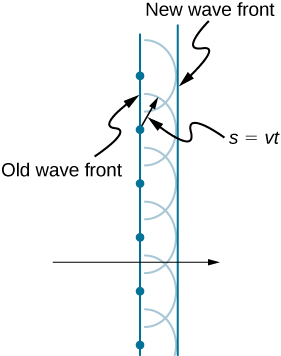### Reflection

(Figure) shows how a mirror reflects an incoming wave at an angle equal to the incident angle, verifying the law of reflection. As the wave front strikes the mirror, wavelets are first emitted from the left part of the mirror and then from the right. The wavelets closer to the left have had time to travel farther, producing a wave front traveling in the direction shown.

Huygens’s principle applied to a plane wave front striking a mirror. The wavelets shown were emitted as each point on the wave front struck the mirror. The tangent to these wavelets shows that the new wave front has been reflected at an angle equal to the incident angle. The direction of propagation is perpendicular to the wave front, as shown by the downward-pointing arrows.### Refraction

The law of refraction can be explained by applying Huygens’s principle to a wave front passing from one medium to another ((Figure)). Each wavelet in the figure was emitted when the wave front crossed the interface between the media. Since the speed of light is smaller in the second medium, the waves do not travel as far in a given time, and the new wave front changes direction as shown. This explains why a ray changes direction to become closer to the perpendicular when light slows down. Snell’s law can be derived from the geometry in (Figure) ((Figure)).

Huygens’s principle applied to a plane wave front traveling from one medium to another, where its speed is less. The ray bends toward the perpendicular, since the wavelets have a lower speed in the second medium.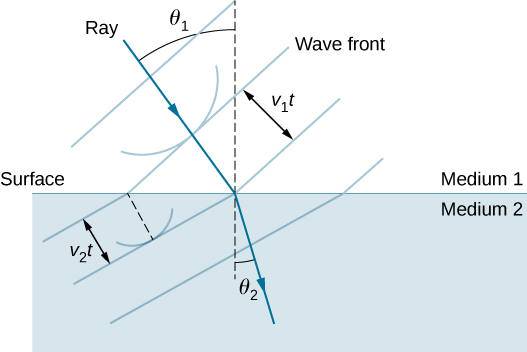Deriving the Law of Refraction By examining the geometry of the wave fronts, derive the law of refraction.

Strategy Consider (Figure), which expands upon (Figure). It shows the incident wave front just reaching the surface at point A, while point B is still well within medium 1. In the timeit takes for a wavelet from B to reach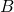on the surface at speed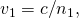a wavelet from A travels into medium 2 a distance of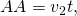whereNote that in this example,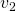is slower than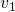because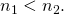Geometry of the law of refraction from medium 1 to medium 2.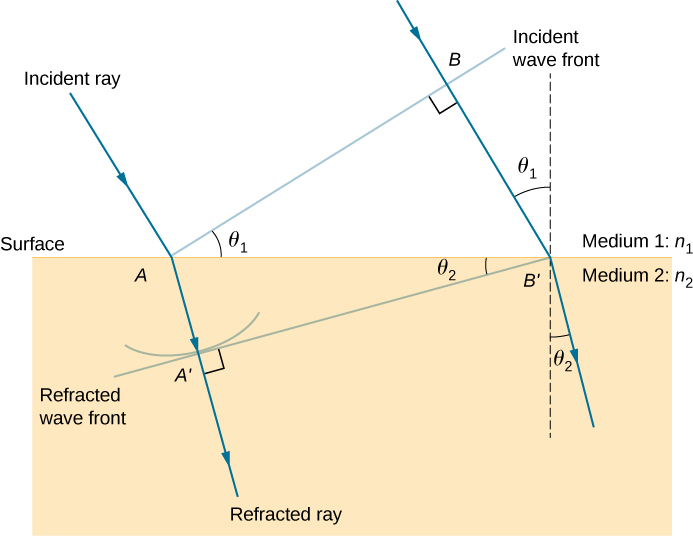Solution The segment on the surface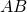is shared by both the triangle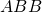inside medium 1 and the triangle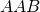inside medium 2. Note that from the geometry, the angle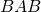is equal to the angle of incidence,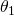. Similarly,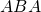is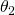.

The length ofis given in two ways as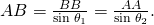Inverting the equation and substituting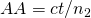from above and similarly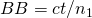, we obtain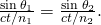Cancellation of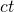allows us to simplify this equation into the familiar form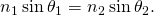Significance Although the law of refraction was established experimentally by Snell and stated in Refraction, its derivation here requires Huygens’s principle and the understanding that the speed of light is different in different media.

Check Your Understanding In (Figure), we had. If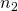were decreased such that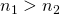and the speed of light in medium 2 is faster than in medium 1, what would happen to the length of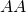? What would happen to the wave front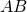and the direction of the refracted ray?becomes longer,tilts further away from the surface, and the refracted ray tilts away from the normal.

This applet by Walter Fendt shows an animation of reflection and refraction using Huygens’s wavelets while you control the parameters. Be sure to click on “Next step” to display the wavelets. You can see the reflected and refracted wave fronts forming.

### Diffraction

What happens when a wave passes through an opening, such as light shining through an open door into a dark room? For light, we observe a sharp shadow of the doorway on the floor of the room, and no visible light bends around corners into other parts of the room. When sound passes through a door, we hear it everywhere in the room and thus observe that sound spreads out when passing through such an opening ((Figure)). What is the difference between the behavior of sound waves and light waves in this case? The answer is that light has very short wavelengths and acts like a ray. Sound has wavelengths on the order of the size of the door and bends around corners (for frequency of 1000 Hz,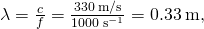about three times smaller than the width of the doorway).

(a) Light passing through a doorway makes a sharp outline on the floor. Since light’s wavelength is very small compared with the size of the door, it acts like a ray. (b) Sound waves bend into all parts of the room, a wave effect, because their wavelength is similar to the size of the door.If we pass light through smaller openings such as slits, we can use Huygens’s principle to see that light bends as sound does ((Figure)). The bending of a wave around the edges of an opening or an obstacle is called diffraction. Diffraction is a wave characteristic and occurs for all types of waves. If diffraction is observed for some phenomenon, it is evidence that the phenomenon is a wave. Thus, the horizontal diffraction of the laser beam after it passes through the slits in (Figure) is evidence that light is a wave. You will learn about diffraction in much more detail in the chapter on Diffraction.

Huygens’s principle applied to a plane wave front striking an opening. The edges of the wave front bend after passing through the opening, a process called diffraction. The amount of bending is more extreme for a small opening, consistent with the fact that wave characteristics are most noticeable for interactions with objects about the same size as the wavelength.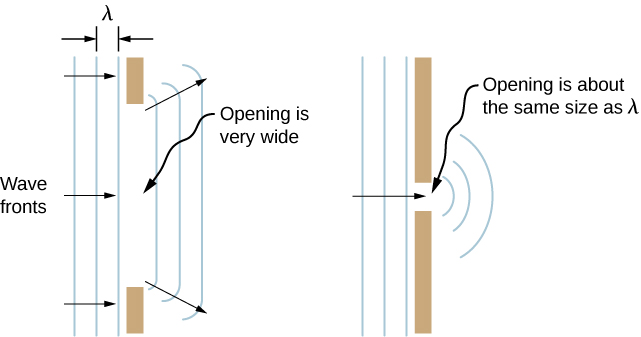### Summary

• According to Huygens’s principle, every point on a wave front is a source of wavelets that spread out in the forward direction at the same speed as the wave itself. The new wave front is tangent to all of the wavelets.
• A mirror reflects an incoming wave at an angle equal to the incident angle, verifying the law of reflection.
• The law of refraction can be explained by applying Huygens’s principle to a wave front passing from one medium to another.
• The bending of a wave around the edges of an opening or an obstacle is called diffraction.

### Conceptual Questions

How do wave effects depend on the size of the object with which the wave interacts? For example, why does sound bend around the corner of a building while light does not?

Does Huygens’s principle apply to all types of waves?

yes

If diffraction is observed for some phenomenon, it is evidence that the phenomenon is a wave. Does the reverse hold true? That is, if diffraction is not observed, does that mean the phenomenon is not a wave?

### Glossary

Huygens’s principle
every point on a wave front is a source of wavelets that spread out in the forward direction at the same speed as the wave itself; the new wave front is a plane tangent to all of the wavelets
wave optics
part of optics dealing with the wave aspect of light